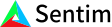1. Bob Anderson
×
IntuitiveML

## Terms: Activation functions

Jun 3, 2020

The original idea behind the activation function is to only propagate signals that are important and ignore signals that aren’t – similar to how neurons in our brain propagate signals. This is why originally, most activation functions looked like this (e.g. a sigmoid curve) – where they are close to zero in the beginning and then all the sudden when they hit some threshold it jumps up close to one.

An activation function is the function that a neuron applies to the weighted sum of its inputs. The neuron basically creates a more general feature from the inputs. Then, with enough of these neurons, we can then construct enough features and then make more general features out of the previous layers’ neurons and so on until we have solved the problem. In image classification, this would be like having the first layer make lines from the pixels, the second layer combine the lines into different shapes, the third combine the shapes into more complicated shapes and so on until we have built up internal representations for cat, mountain, tree, etc.

In the intro I said that most activation functions looked like this in the beginning. In reality, activation functions usually only need two properties: they are differentiable and nonlinear. This means that they can have all sorts of shapes! We want the function to be differentiable so that we can use backpropagation or another gradient based method of learning, but I will talk more about that in a future video.

We don’t usually want to use a linear activation function because with a linear activation function, regardless of how many layers or neurons you use the final function will be always be a linear combination of the inputs. Remember, we assume that there is some actual function that describes how to solve our problem. If we only use linear combination, we are limiting what our function can be, whereas if we use a nonlinear activation function then we can guarantee that it is possible to approximate our actual function – assuming that we use enough layers and nodes.